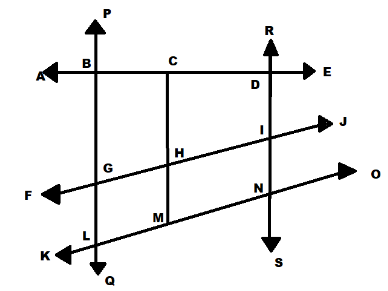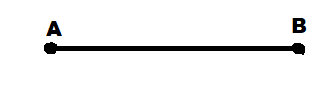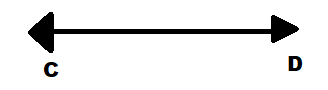QuestionAnswers

# With respect to the figure, name the five line segmentsA) ND, GI, LN, BL, CMB) ND, GI, LN, BM CMC) ND, GI, BN, BM, CMD) ND, GI, BN, BL, CMHint: A line segment is a line having two endpoints so we will find all such lines which have two end points and then check each of the options and observe whether they are true or false.

We now that a line segment is a line having two endpoints
Hence all such lines having two end points are:-
BL, BG, CH, CM, DI, IN, BC, BD, LM, LN, GH, GI, DN, GL, HM, MN, HI ……………………….(1)
Now let us check for all the options
A) ND, GI, LN, BL, CM
According to the above stated line segments in (1)
ND is a line segment.
GI is a line segment.
LN is a line segment.
BL is a line segment.
CM is a line segment.
Hence option A is correct.
B) ND, GI, LN, BM CM
According to the above stated line segments in (1)
ND is a line segment.
GI is a line segment.
LN is a line segment.
BM is not a line segment.
CM is a line segment.
Hence option B is incorrect.
C) ND, GI, BN, BM, CM
According to the above stated line segments in (1)
ND is a line segment.
GI is a line segment.
BN is not a line segment.
BM is not a line segment.
CM is a line segment.
Hence option C is incorrect.
D) ND, GI, BN, BL, CM
According to the above stated line segments in (1)
ND is a line segment.
GI is a line segment.
BN is not a line segment.
BL is a line segment.
CM is a line segment.
Hence option D is incorrect.

Hence the answer is option A.

Note: Students might make a mistake in determining the line segment and a line.
They should note that a line segment has two endpoints while a line does not have any end point.
A line segment is represented as:A line is represented as:View Notes
CBSE Class 6 Maths Chapter 3 - Playing with Numbers FormulasNCERT Books Class 6 English a Pact with the SunNumber Name 1 to 50CBSE Class 6 to 12 Maths Formulas - Important Math FormulasTable of 6 - Multiplication Table of 6CBSE Class 6 Maths FormulasCBSE Class 6 Maths Chapter 11 - Algebra FormulasCBSE Class 6 Maths Chapter 6 - Integers FormulasCBSE Class 6 Maths Chapter 10 - Mensuration FormulasNCERT Class 6 Maths Book PDFImportant Questions for CBSE Class 6 English A Pact with The Sun Chapter 6 - The Monkey and the CrocodileImportant Questions for CBSE Class 6 English A Pact with The Sun Chapter 9 - What Happened to The ReptilesImportant Questions for CBSE Class 6 English A Pact with The SunImportant Questions for CBSE Class 6 English A Pact with The Sun Chapter 8 - A Pact with the SunImportant Questions for CBSE Class 6 English A Pact with The Sun Chapter 2 - The Friendly MongooseImportant Questions for CBSE Class 6 English A Pact with The Sun Chapter 3 - The Shepherd's TreasureCBSE Class 6 Science Fun with Magnets WorksheetsImportant Questions for CBSE Class 6 English A Pact with The Sun Chapter 4 - The Old Clock ShopImportant Questions for CBSE Class 6 English A Pact with The Sun Chapter 1 - A Tale of Two BirdsImportant Questions for CBSE Class 6 English A Pact with The Sun Chapter 5 - TansenCBSE Previous Year Question Papers Class 12 Maths with SolutionsCBSE Previous Year Question Papers Class 10 Maths with SolutionsCBSE Class 12 Maths Question Paper 2018 with Solutions - Free PDFCBSE Class 10 Maths Question Paper 2019 with Solutions - Free PDFCBSE Class 12 Maths Question Paper 2017 with Solutions - Free PDFCBSE Class 12 Maths Question Paper 2019 with Solutions - Free PDFCBSE Class 12 Maths Question Paper 2015 with Solutions - Free PDFCBSE Class 10 Hindi A Question Paper with SolutionsCBSE Class 10 English Communicative Question Paper with SolutionsCBSE Class 10 Hindi B Question Paper with SolutionsNCERT Solutions for Class 6 English A pact with the Sun Chapter-6RS Aggarwal Class 6 Solutions Chapter-11 Line Segment, Ray and LineNCERT Solutions for Class 6 English A pact with the SunRD Sharma Class 6 Solutions Chapter 6 - Fractions (Ex 6.6) Exercise 6.6RS Aggarwal Solutions Class 6 Chapter-11 Line Segment, Ray and Line (Ex 11A) Exercise 11.1RD Sharma Class 6 Solutions Chapter 6 - Fractions (Ex 6.3) Exercise 6.3RD Sharma Class 6 Solutions Chapter 6 - Fractions (Ex 6.9) Exercise 6.9NCERT Solutions for Class 6 Maths Chapter 3RS Aggarwal Solutions Class 6 Chapter-6 Simplification (Ex 6B) Exercise 6.2RD Sharma Class 6 Solutions Chapter 6 - Fractions (Ex 6.7) Exercise 6.7